Our World Statistics Day conversations have been a great reminder of how much statistics can inform our lives. Do you have an example of how statistics has made a difference in your life? Share your story with the Community!
Choose Language Hide Translation Bar
Highlighted

## Running average by data series using For Loop and same script on multiple columns

Hello, I need to get the average for all the columns and sort it by the column header. The data is attached in a jmp file that imports from excel! Thanks!

2 ACCEPTED SOLUTIONS

Accepted Solutions
Highlighted

## Re: Running average by data series using For Loop and same script on multiple columns

@tylerram123
Something like this

``````Clear Log() ; Clear Globals();

// Script to Open File
FilePath = "C:\RC23.xlsx";  // Edit to have your custom File Path
dt_Input = Open(
FilePath,
Worksheets( "RC23" ),
Use for all sheets( 1 ),
Concatenate Worksheets( 0 ),
Create Concatenation Column( 0 ),
Worksheet Settings(
1,
Number of Rows in Headers( 1 ),
Headers Start on Row( 2 ),
Data Starts on Row( 3 ),
Data Starts on Column( 1 ),
Data Ends on Row( 0 ),
Data Ends on Column( 0 ),
Replicated Spanned Rows( 1 ),
Suppress Hidden Rows( 1 ),
Suppress Hidden Columns( 1 ),
Suppress Empty Columns( 1 ),
Treat as Hierarchy( 0 ),
Multiple Series Stack( 0 ),
Import Cell Colors( 0 ),
Limit Column Detect( 0 ),
Column Separator String( "-" )
)
);

// Method 1
dt_Method1 = dt_Input << Summary(
Mean( :Column 1 ),
Mean( :Ic 2 ),
Mean( :RnN 2 ),
Mean( :RnP 2 ),
Mean( :Rn 2 ),
Mean( :IcN 3 ),
Mean( :IcP 3 ),
Mean( :Ic 3 ),
Mean( :RnN 3 ),
Mean( :RnP 3 ),
Mean( :Rn 3 ),
Mean( :IcN 4 ),
Mean( :IcP 4 ),
Mean( :Ic 4 ),
Mean( :RnN 4 ),
Mean( :RnP 4 ),
Mean( :Rn 4 ),
Mean( :IcN 5 ),
Mean( :IcP 5 ),
Mean( :Ic 5 ),
Mean( :RnN 5 ),
Mean( :RnP 5 ),
Mean( :Rn 5 ),
Mean( :IcN 6 ),
Mean( :IcP 6 ),
Mean( :Ic 6 ),
Mean( :RnN 6 ),
Mean( :RnP 6 ),
Mean( :Rn 6 ),
Freq( "None" ),
Weight( "None" ),
statistics column name format( "stat of column" )
);

// Method 2
dt_Method2 = (dt_Input << Tabulate(
Row Table(
Analysis Columns(
:Ic 2,
:RnN 2,
:RnP 2,
:Rn 2,
:IcN 3,
:IcP 3,
:Ic 3,
:RnN 3,
:RnP 3,
:Rn 3,
:IcN 4,
:IcP 4,
:Ic 4,
:RnN 4,
:RnP 4,
:Rn 4,
:IcN 5,
:IcP 5,
:Ic 5,
:RnN 5,
:RnP 5,
:Rn 5,
:IcN 6,
:IcP 6,
:Ic 6,
:RnN 6,
:RnP 6,
:Rn 6
),
Statistics( Mean )
)
)
)) << Make Into Data Table;``````
Best
Uday
Highlighted

## Re: Running average by data series using For Loop and same script on multiple columns

Below is a script that create the summary output table you stated was what you wanted.  The script goes from the JMP data table you provided, through to it's completion.  About 80% of the script was actually created by running the steps interactively in JMP, and then just having JMP save the script it used for the interactive processing to a script window.  All of the steps and functions used in the script are documented in the Scripting Guide and Scripting Index

Help==>Books==>Scripting Guide

Help==>Scripting Guide

``````Names Default To Here( 1 );
dt = Current Data Table();

// Correct the data table using the JMP table imported from the Excel file
For( i = 2, i <= N Cols( dt ), i++,
Column( dt, i ) << set name(
Word( 1, Column( dt, i ) << get name, " " ) || " " || Column( dt, i )
)
);

dt << delete rows( 1 );

For( i = 2, i <= N Cols( dt ), i++,
Column( dt, i ) << data type( numeric ) << modeling type( continuous )
);

// Change the name of the first column to H(Oe)
Column( dt, 1 ) << set name( "H(Oe)" );

// Create a list of all numeric column names
colNamesList = dt << get column names( numeric );

// Summarize the data
dtSum = dt << Summary(
Mean( Eval( colNamesList ) ),
Freq( "None" ),
Weight( "None" ),
statistics column name format( "column" ),
Link to original data table( 0 )
);

// Create final table and sort it in alphabetical order

// First delete the unneeded column N Rows
dtSum << delete columns( "N Rows" );

dtFinal = dtSum << Transpose(
columns( Eval( colNamesList ) ),
Label column name( "Column" ),
Output Table( "Summary " )
);

// Change the name of the "Row 1" column to "Mean"
dtFinal:Row 1 << set name( "Mean" );

// Sort the data table
dtFinal = dtFinal << sort( by( Column ), order( Ascending ), Replace Table( 1 ) );``````
Jim
13 REPLIES 13
Highlighted

## Re: Running average by data series using For Loop and same script on multiple columns

@tylerram123,
When you imported the data, it would be helpful if you made the first row that you have as your column name. If that happens, then you can use the Tabulate function:

You may use the drag and drop feature to compute different statistics as is shown. As to where you find tabulate, that is shown in the screenshot below: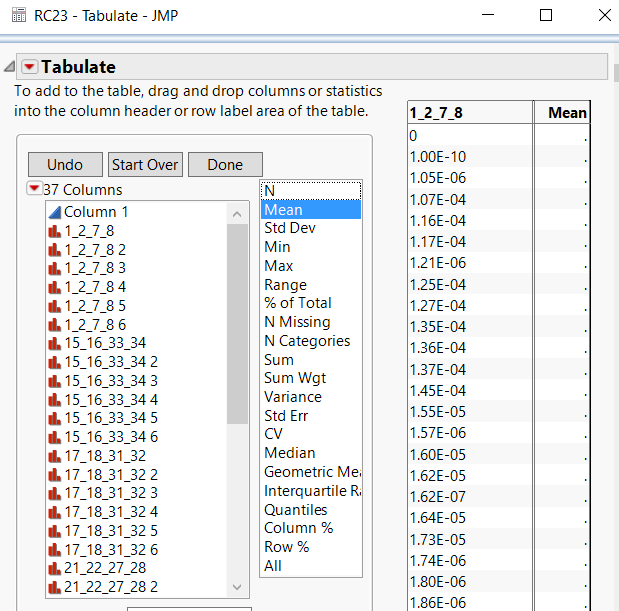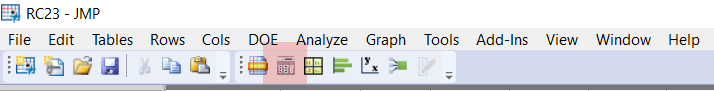Best
Uday
Highlighted

## Re: Running average by data series using For Loop and same script on multiple columns

Is there a way to do this by scripting?

Highlighted

## Re: Running average by data series using For Loop and same script on multiple columns

@tylerram123
Something like this

``````Clear Log() ; Clear Globals();

// Script to Open File
FilePath = "C:\RC23.xlsx";  // Edit to have your custom File Path
dt_Input = Open(
FilePath,
Worksheets( "RC23" ),
Use for all sheets( 1 ),
Concatenate Worksheets( 0 ),
Create Concatenation Column( 0 ),
Worksheet Settings(
1,
Number of Rows in Headers( 1 ),
Headers Start on Row( 2 ),
Data Starts on Row( 3 ),
Data Starts on Column( 1 ),
Data Ends on Row( 0 ),
Data Ends on Column( 0 ),
Replicated Spanned Rows( 1 ),
Suppress Hidden Rows( 1 ),
Suppress Hidden Columns( 1 ),
Suppress Empty Columns( 1 ),
Treat as Hierarchy( 0 ),
Multiple Series Stack( 0 ),
Import Cell Colors( 0 ),
Limit Column Detect( 0 ),
Column Separator String( "-" )
)
);

// Method 1
dt_Method1 = dt_Input << Summary(
Mean( :Column 1 ),
Mean( :Ic 2 ),
Mean( :RnN 2 ),
Mean( :RnP 2 ),
Mean( :Rn 2 ),
Mean( :IcN 3 ),
Mean( :IcP 3 ),
Mean( :Ic 3 ),
Mean( :RnN 3 ),
Mean( :RnP 3 ),
Mean( :Rn 3 ),
Mean( :IcN 4 ),
Mean( :IcP 4 ),
Mean( :Ic 4 ),
Mean( :RnN 4 ),
Mean( :RnP 4 ),
Mean( :Rn 4 ),
Mean( :IcN 5 ),
Mean( :IcP 5 ),
Mean( :Ic 5 ),
Mean( :RnN 5 ),
Mean( :RnP 5 ),
Mean( :Rn 5 ),
Mean( :IcN 6 ),
Mean( :IcP 6 ),
Mean( :Ic 6 ),
Mean( :RnN 6 ),
Mean( :RnP 6 ),
Mean( :Rn 6 ),
Freq( "None" ),
Weight( "None" ),
statistics column name format( "stat of column" )
);

// Method 2
dt_Method2 = (dt_Input << Tabulate(
Row Table(
Analysis Columns(
:Ic 2,
:RnN 2,
:RnP 2,
:Rn 2,
:IcN 3,
:IcP 3,
:Ic 3,
:RnN 3,
:RnP 3,
:Rn 3,
:IcN 4,
:IcP 4,
:Ic 4,
:RnN 4,
:RnP 4,
:Rn 4,
:IcN 5,
:IcP 5,
:Ic 5,
:RnN 5,
:RnP 5,
:Rn 5,
:IcN 6,
:IcP 6,
:Ic 6,
:RnN 6,
:RnP 6,
:Rn 6
),
Statistics( Mean )
)
)
)) << Make Into Data Table;``````
Best
Uday
Highlighted

## Re: Running average by data series using For Loop and same script on multiple columns

How would I export that to a Journal?

Highlighted

## Re: Running average by data series using For Loop and same script on multiple columns

You can do that easily by doing the following:

``````dt << Journal();
// in your case dt_Method1 ``````

However, I would advice you to kindly put all your requests at once or as separate questions

Best
Uday
Highlighted

## Re: Running average by data series using For Loop and same script on multiple columns

Is this the result you want?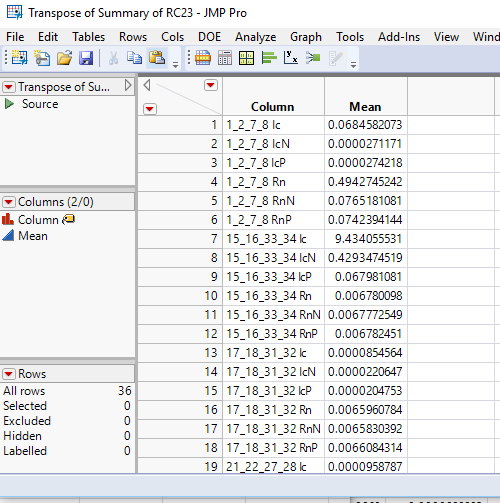I could not tell.  The Excel file was improperly input if the above result is what you want.  The Excel Import Wizard or the JMP Addin for Excel,  will allow you to indicate what row the column headers are on, and what what row the data starts on.  Or, you can cut and paste the data from Excel into JMP.  But the way you imported the data placed what I assume was the second line of the column header as the first row of the data.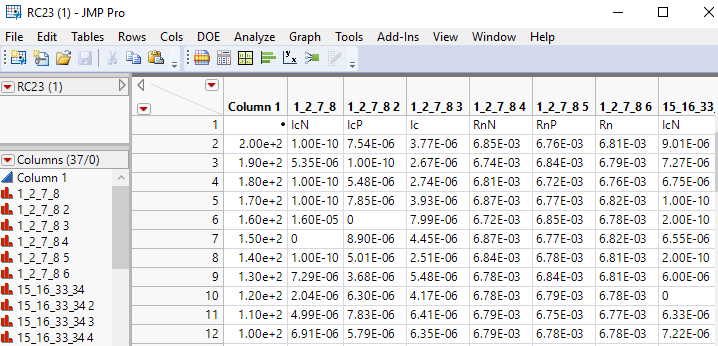The result of this, was in order for JMP to read in your data, it had to assume that all of the columns, except the first column contained a non numeric value, and therefore the column had t be read in as a numeric column.  The JMP data table is an analysis table structure, not a cell based structure.  All data in a given cell must be of the same type....for your data, either character or numeric.

So, the first thing for you to do, is to correct the import of your data.

If you used the JMP Excel Addin, use the Preferences icon at the top of the screen to set the Header Rows.

If you are opening the Excel file from a

File==>Open

in JMP, single click on the Excel file you want to open, and then go to the down arrow next to the "Open" button on the window, and select"Use Excel Wizard"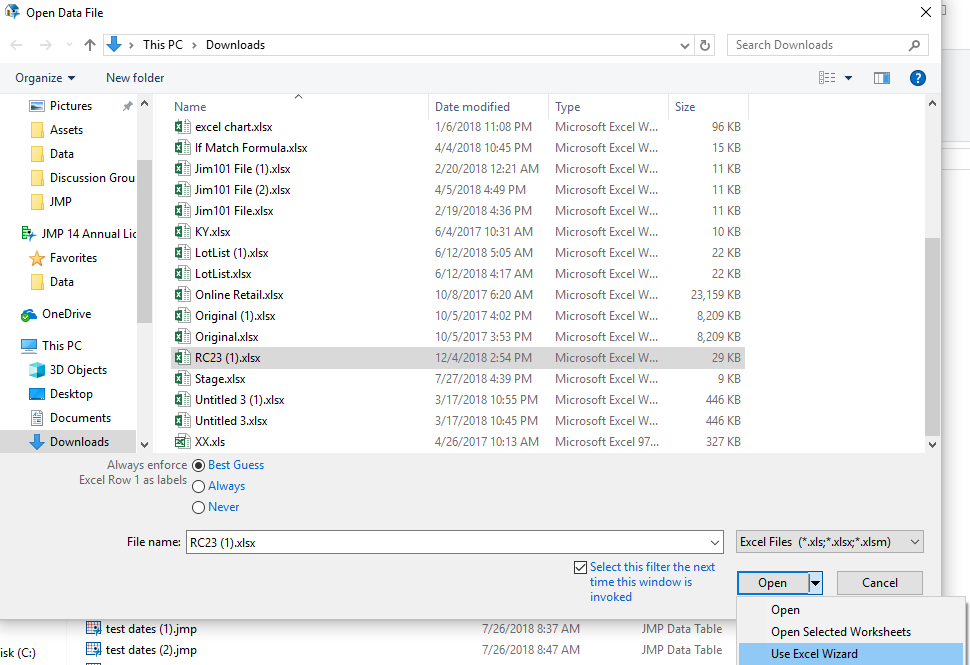It will guide you through the proper importing of your Excel file.

Once that is done, it is a simple mater of using the Summary table platform

Jim
Highlighted

## Re: Running average by data series using For Loop and same script on multiple columns

The first picture is what I am looking for. You are right, for some reason it did not put in the column header for Column 1 which should be H (Oe). Do you know how to do what you did for the first picture using SAS scripting? Thanks again for the help!

Highlighted

## Re: Running average by data series using For Loop and same script on multiple columns

To be clear.....do you want the solution in the SAS language, or in the JMP Scripting Language? Both the SAS System and JMP are products offered from SAS Institute, but are vastly different in syntax.
Jim
Highlighted

## Re: Running average by data series using For Loop and same script on multiple columns

I wanted JMP scripting. Sorry for the ambiguity!

Article Labels

There are no labels assigned to this post.